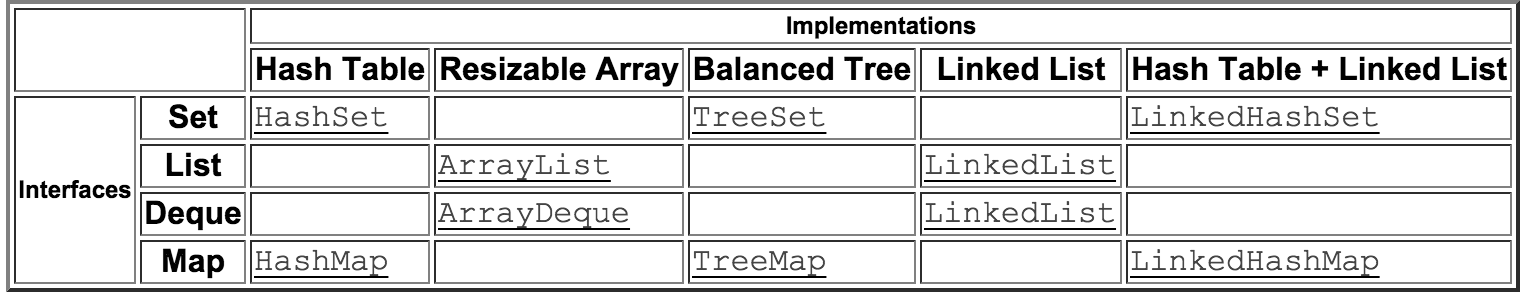By

# Why study DS?

1. Search, Organize and Manipulate information
2. Reduces complexity
3. Efficiency in solving algorithms

## Terms, Definitions & Properties

1. Linear Vs Non Linear
2. Abstract Data type
3. Time, Space Complexity & Big O Notation
4. Ordering in a data structure
5. Mutable vs Immutable
6. Concurrent
7. Duplication

## Big (O) notation

Growth rate of a function or a program

``````    # O(n)
a = [1..1000]
a.each { |n| print n}
``````
``````    # O(n^2)
a,b = (1..10),(1..10)
a.each do |m|
b.each do |n|
p m*n
end
end
``````
``````    # O(n!) All possible permuattions of a string
"ruby".chars.to_a.permutation.map &:join
=> ["ruby", "ruyb", "rbuy", "rbyu", "ryub", "rybu", "urby", "uryb", "ubry", "ubyr", "uyrb", "uybr", "bruy", "bryu", "bury", "buyr", "byru", "byur", "yrub", "yrbu", "yurb", "yubr", "ybru", "ybur"]
``````

## Big (O) continued

Classic example Binary Search in sorted array

``````    # O(log(n))
class Array
def binary_search(val, low=0, high=(length - 1))
return nil if high < low
mid = (low + high) / 2
case
when self[mid] > val then binary_search(val, low, mid-1)
when self[mid] < val then binary_search(val, mid+1, high)
else mid
end
end
end
``````
``````    # O(2^n) Recursive or Naive Fibonacci
def fibonacci(n)
n <= 1 ? n :  fibonacci( n - 1 ) + fibonacci( n - 2 )
end
``````

1. Insert
2. Search
3. Retrieve
4. Space Used

Handy Cheatsheet

## Common DS

• Array
• Hash, Bloom Filter
• Stack, Queue, Dequeue
• Set, BitArray
• Tree, Binary Search Tree, Heap, Trie
• Graph

## DS in Ruby Core & Std

• Array
• Hash
• Set, SortedSet
• Matrix
• Vector
• Range

## DS in Java

Java Collections FrameworkExtensive use of DS not in Java Core

Enough DS to choose from that there is a flowchart for selecting the right one.

## Why DS not as distinct in Ruby

Ruby's API blends queues, stacks, sets, arrays and lists in a few classes, for a richer api.

# Hash

• Key/Vaue Pair
• O(1) insert, search, delete
• Internally an array
• Uses a hash function & division method to fit data into an array

## How it works

How the Hash works in Ruby

## How to implement one in Ruby

Hash implementation

## Implementing DS in Ruby

• Understand Ruby API's and implementation better
• A nice refresher/ academic exercise
• Discover libraries

Let's start

## Stack

• FIFO
• Operations supported
• push
• pop
• peek
• Implementation varies
• Array

## Queue

• LIFO
• Operations supported
• shift
• unshift
• Implementation varies
• Array

# Set

• Unordered elements
• Distinct elements
• Tests for membership/inclusion not necessarily retrieval
• No set in Core till 1.9.3
• MRI Ruby implemented as a Hash
• Faster then [1,2,3].uniq! since it is just a Hash
• No literal notation, use Set.new
• The expected operations in a Set -member? -union | -intersection & -difference - -xor ^
• Used in rails for routing, caching etc.

# SortedSet

• Same properties of Set but ordered elements
• MRI Ruby implements natively in C with a Red-Black Tree DS
• Sets in Java are also implemented in a similiar way.
• Java 's TreeSet ~ Ruby's SortedSet
• Java 's HashSet ~ Set

# CustomSet

• Internally stored as an array
• Supports instantiation with any enumerable type
• Includes Enumerable and implements `each` to get some methods for free
• Return types should enable chaining

Implementation

# MultiSet

• members allowed to appear more than once.
• `{a, a, b}`
• the order of elements is irrelevant: The multisets
• {a, a, b} and {a, b, a} are equal.
• it provides the count or multiplicity of an element in the set.
• `count (a) = 2`
• it can return the distinct elements of the set.
• `{a, b}`
• it returns the cardinality of the set, total number of elements including duplicates.
• `6`

Implementation

# BitArray

• Set like DS, but dense packed bits
• Implemented using an array as storage

Implementation

# Bloom Filter

• Check membership in a set
• Always gives a right answer if element is not present
• false positive if present
• Great for disk I/O
• Safe browsing
• Mix of hash & Set
• uses multiple hash functions to verify membership

# Circular Buffer

• Implemented using an array as storage
• Overwrites after full

# Tree

• Hierarchical DS
• n-Ary tree
• Binary Tree
• Binary Search Tree
• Heap
• Trie
• quick terminolgy
• root
• leaf
• level
• path
• siblings
• Real world usage, File system, RDBMS

# BST

• Binary tree with ordering
• left < right
• no duplicates,
• Search/Insert/delete O(log n)

# Heap

• Binary tree
• Max/Min at root
• No order left/right
• Max heap, min heap

# Trie

• A tree with prefix stored
• Space efficient when there is repetition
• Example storing urls
``````     www -> meetup -> com -> ruby -> sv ->
-> java
-> hiking
-> net
``````

# Matrix

• Creating multi dimensional arrays in Ruby
``````      a =[][]
a = Array.new() {} n dimensional matrix
``````
``````  Matrix.build(3, 3) {|row, col| 0 }
=> 0, 0, 0
0, 0, 0
0, 0, 0
``````

# Graphs & other DS

• Graphs
• Singly linked list is a basic graph
• Tree with loops
• Sparse Matrices
• Space Partitioning Trees
• K-D tree